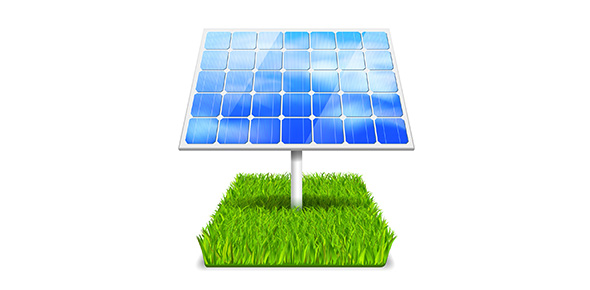# Electical Energy

11 Questions | Total Attempts: 87SettingsElectrical energy quiz

Related Topics
• 1.
Name the 3 components of circuits (3 answers)
• A.

The rate at which electrical energy is converted to another form of energy.

• B.

A circuit breaker is another device that prevents a circuit from overheating and causing a fire.

• C.

A source of voltage difference that can be provided by a battery.

• D.

One or more devices that uses electrical energy.

• E.

Conductors such as wires that connect he devices to the source of voltage difference to form a closed path.

• 2.
Circuit breaker
• A.

The rate at which electrical energy is converted to another form of energy,

• B.

Device that prevents a circuit from overheating and causing a fire.

• C.

Devices that use electrical energy.

• D.

Metal that melts if the current becomes too high.

• 3.
T  or   FTo use electrical energy, a complete circuit must be made.
• A.

True

• B.

False

• 4.
What are the two kinds of circuits?
• A.

Series (open)

• B.

Closed

• C.

Electric

• D.

Parallel

• E.

Fuse

• 5.
The circuit has only one loop to flow through.
• A.

Series

• B.

Closed

• C.

Parallel

• D.

Open

• E.

Fused

• 6.
T  or FWhen any part of a series circuit is discontinued, most of the currency flows through the circuit.
• A.

True

• B.

False

• 7.
T  or FOpen circuit is the same as series circuit because the parts of a series circuit are wired one after another thus the amount of current is the same through every part.
• A.

True

• B.

False

• 8.
Contains two or more branches for current to flow through.
• A.

Series

• B.

Open

• C.

Parallel

• D.

Fuses

• 9.
T  or FAccording to Ohms Law, more currents flow through the branches that have higher resistance.
• A.

True

• B.

False

• 10.
Contains a small piece of metal that melts if the current becomes too high.
• A.

Series

• B.

Open

• C.

Parallel

• D.

Fuses

• 11.
T  or FMelting of a fuse causes a break in the circuit, stopping the flow of current through the over circuit.
• A.

True

• B.

False